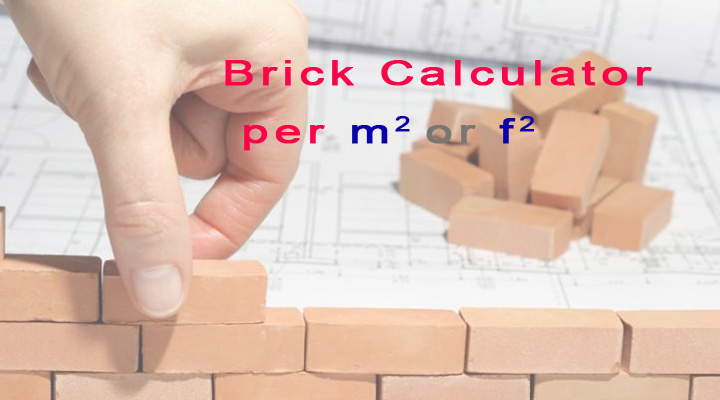# Brick Calculator – Bricks Per Square Meter or Bricks Per Square Feet

If you want to know how many bricks needed per square meter or square feet, in this post we are going to discuss brick quantity calculation. You can use this brick quantity calculator formula for your house or wall construction.

The standard co-ordinating size for brickwork is 225 mm x 112.5 mm x 75 mm (length x depth x height). This includes 10 mm mortar joints, and so actual size of a common brick is 215 x 102.5 x 65 mm. There are varieties of bricks such as common bricks, facing bricks, engineering bricks, modular, queen, jumbo, closure, king, norman, roman and utility bricks.

## Number of bricks for 1 Cubic meter brickwork

Standard dimensions of brick in metric units are 225 x 112.5 x 75 mm.

1. multiply these dimensions to get the volume of a brick, 0.225 m x 0.1125 m x 0.075 m=0.00189 cubic meter.
2. In one cubic meter, number of bricks will be (1/0.00189)=529.1 bricks.
3. 10% of brickwork will be covered by mortar.
4. Subtract 10% bricks from the 529.1, you will get 529.1-52.91=476.20 bricks.
5. Add 5% wastage of bricks.
6. 5% of 476.20 is 23.80 bricks.
7. Add 23.80 and 476.20 to get number of bricks in one cubic meter brickwork.
8. 23.80+476.20=500 bricks.## Number of bricks for 1 Cubic feet brickwork

1. The standard dimensions of brick is 9 inch x 3 inch x 4.5 inch.
2. Convert these dimensions into feet.
3. Now multiply these dimensions to get volume of a brick.
4. 0.75 feet x 0.25 feet x 0.375 feet = 0.0703125 cubic feet.
5. Bricks required for 1 cubic feet brickwork will be (1/0.070) = 14.285 bricks
6. 10% space of brickwork is covered by mortar.
7. Subtract 10% bricks from 14.285, 14.285-1.4285=12.85 bricks.
8. Add 5% wastage of bricks.
9. 5% of 12.85 is 0.64 bricks.
10. Add 0.64 and 12.85 to get number of bricks in 1 cubic feet, you get 0.64 + 12.85 =13.492 bricks or you can say that 13.5 bricks.

## Number of bricks in 100 Cubic feet brickwork

As you know that there are 13.5 bricks in 1 cubic feet. So in 100 cubic feet there will be 1350 bricks.

## Bricks per square foot

### Bricks per square foot

4.5 inch 5.0625 bricks
9 inch 10.125 bricks
13.5 inch 15.1875 bricks

## Bricks per square meter

### Bricks per square meter

4.5 inch 57.16 bricks
9 inch 114.329 bricks
13.5 inch 171.49 bricks

## Bricks calculation formula

Bricks calculation formula is written below.

### In Feet

• Length of wall in feet x Height of wall in feet x Thickness of wall in feet x 13.5 = number of bricks

### In meter

• Length of wall in meter x Height of wall in meter x Thickness of wall in meter x 500 = number of bricks

## How to calculate bricks for a wall?

To calculate bricks in a wall, you need to know dimensions of the wall construction. For example, if a wall is 10 feet long with 10 feet height and 9-inch thickness. Then you can find its bricks needed for the construction.

Multiple dimensions of wall. 10 feet x 10 feet x 0.75 feet. You will get 75 cubic feet. You have seen above that there are 13.5 bricks in 1 cubic feet brickwork. So there will be 75 x 13.5 =1012.5 bricks in 75 cubic feet. In this method, you can calculate bricks for any wall.